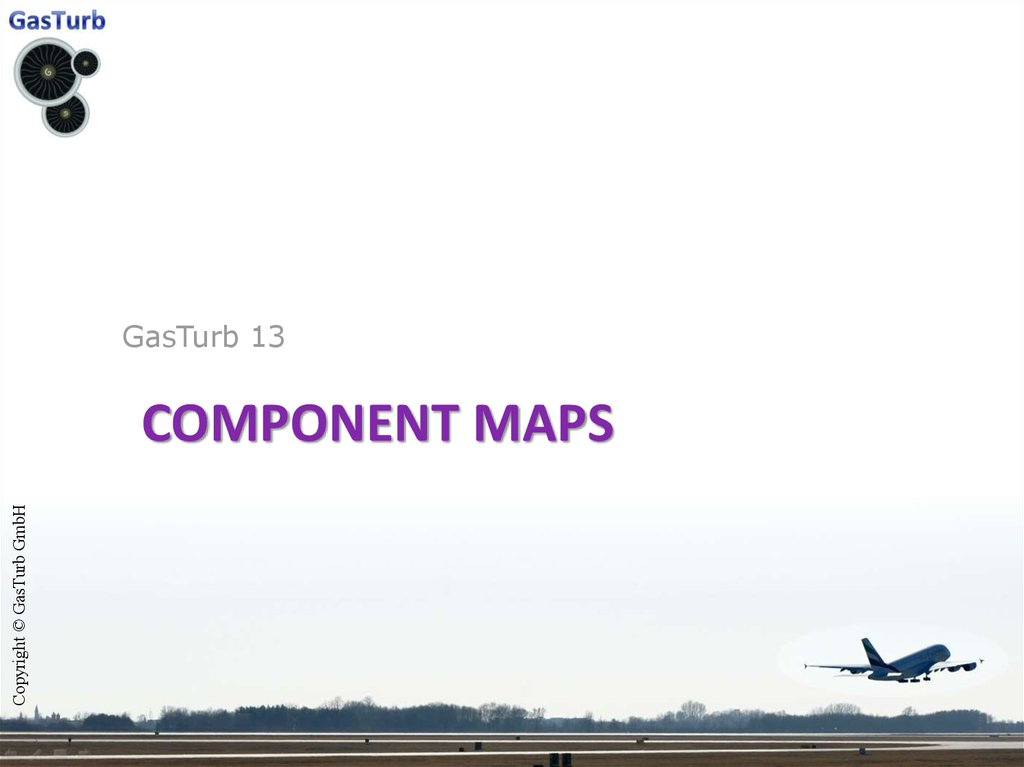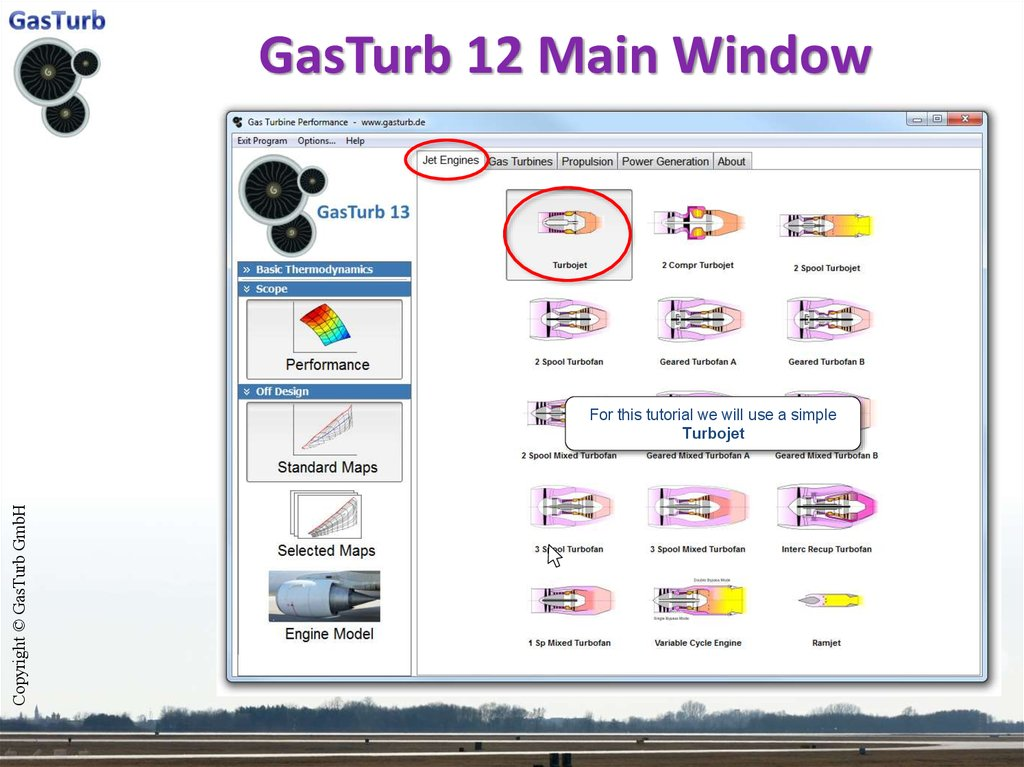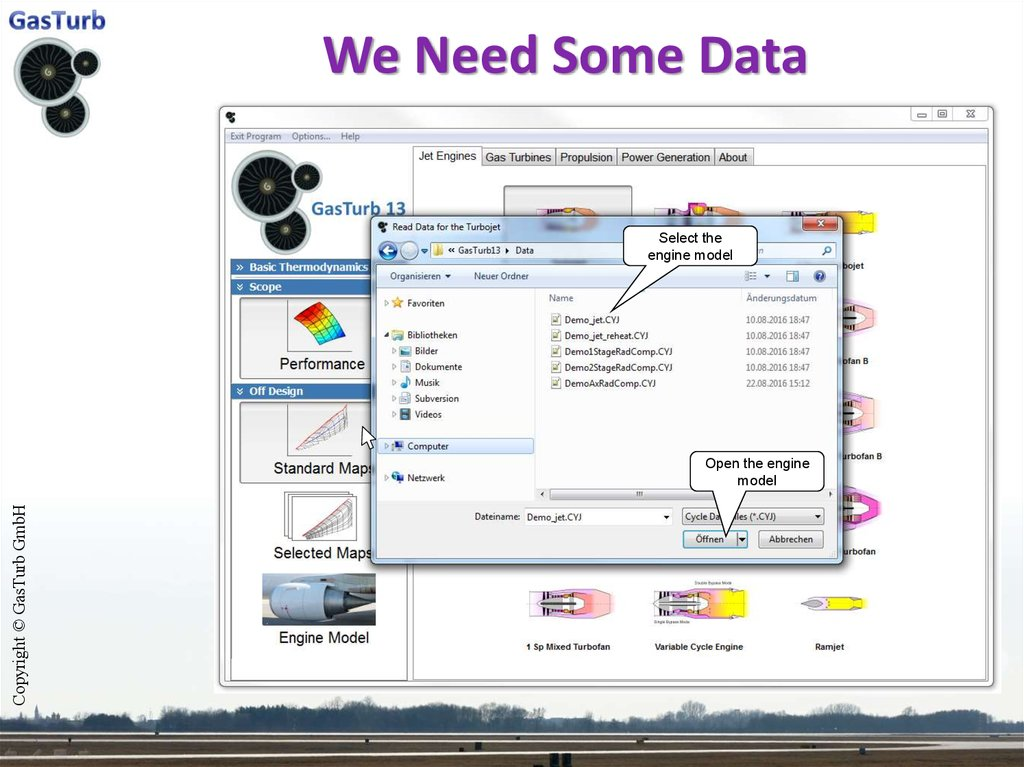# Component maps

GasTurb 13
COMPONENT MAPS

## 2. GasTurb 12 Main Window

For this tutorial we will use a simple
Turbojet

## 3. We Need Some Data

Select the
engine model
Open the engine
model

Run an Off-Design
simulation without
changes

## 5. Off-Design Point Summary

Have a lock at the
compressor map
This is the cycle design point,
calculated in off-design mode.

## 6. Standard Compressor Map

The circle marks the cycle
design point, the yellow
square marks the off-design
operating point.
Close the result
window
W2RStd = Mass
Flow Corrected to
Standard Day
Conditions
The cycle design point is
In this special Mass
case –Flow
because
we have
W2RStd
= 32 calculated
kg/s
Pressure
= mode
12
the cycle design
point inRatio
off-design
– the
0.85
yellowEfficiency
square is inside the=circle.

Click on Special to
configure Special
Maps
The Standard Maps yield in many cases
reasonable trends. However, for accurate
simulations the Standard Maps must be replaced
by Special Maps.

## 8. HP Compressor Map

In this
window
youcoordinates
You can
either
edit the
of the
appropriate
map from
design
point orcompressor
move the design
pointfile.
with
the mouse: click it, keep the button
To shorten
theit.tutorial we do not read a
pressed,
move
special map, we stay with the default map.
We will set the cycle design point now to
Beta,ds
= 0.4
N/sqrt(T),ds = 1.05
At the yellow square the
pressure
ratio inthe
theefficiency
map is 8.3138.
At the yellow square
in the map
To scale the mapisin0.86015.
such a way that it fits to
the
point
(P/P=12)
factor
Tocycle
scaledesign
the map
in such
a waythe
that
it fitsfP/P-1
to
=
1.504
is
applied:
the cycle design point ( =0.85) the factors f =
0.9884 and fReynolds=0.9998 are applied:
P / Pscaled is the
1 highest
f P / P 1 * efficiency
P / Pmap found
1
Peak Efficiency
in
f
*
f
*
scaled
Re
ynolds
map
the
scaled
map.
The coordinates of the design point in the map
(the yellow square) are
Beta,ds = 0.5 and N/sqrt(T),ds = 1
The yellow
square marks
the cycle design
point.

## 9. Map Scaling

Close the map
window
At the yellow square the efficiency in the map
is now 0.8246.
To scale the map in such a way that it fits to
the cycle design point ( =0.85) the factors f =
Peak1.03
Efficiency
is the=0.9998
highest are
efficiency
found
and fReynolds
applied:
in the scaled map. Previously it was 0.8568,
scaled now
f *it isf Re0.8929.
ynolds * map
The reason for that is: the cycle design point
efficiency remains always 0.85

## 10. Save the data as Engine Model

Click on Operating
Line
Next we run a
single operating line
with the default
settings.
The data can only be stored as Engine Model
File if all data on the Steady State page are
identical to those from the cycle design point
and if all the Modifiers are zero.
An Engine Model File contains in addition to a
normal data file information about the
component maps and how they are scaled.

Select Compr
Run the Operating
Line
Select No

## 12. Compressor Map New

If we would use the Standard Map with the
default setting of the cycle design point
(i.e. Beta,ds = 0.5 and N/sqrt(T),ds = 1),
Peak Efficiency is
then the operating line in the compressor
the
map would look as in the next slide. highest efficiency
found in the map, it
is 0.8929.
The speed line passing through the cycle
design point has the relative corrected speed
value of 1.
The numbers at the other speed lines are
those from the un-scaled map, divided by
N/sqrt(T),ds = 1.05

## 13. Compressor Map Old

Peak Efficiency is
the highest efficiency
found in the map,
here it is 0.8568.
The speed line passing through the cycle
design point has the relative corrected speed
value of 1.

## 14. Effect of Map Scaling on SFC

Hint:
This slide is made of two pictures which
were copied to the clipboard from GasTurb
and then pasted into Power Point.
Standard Map Scaled With the Special Design
Point Coordinates
Beta,ds = 0.4 and N/sqrt(T),ds = 1.05
Standard Map Scaled With the Default Design
Point Coordinates
Beta,ds = 0.5 and N/sqrt(T),ds = 1
This is the cycle design
point where both
operating lines agree.

## 15. Comparing Measured Data With GasTurb

And run another
Operating line
Switch back to
Standard maps

Run the Operating
Line
demo_jet.tst
measured data
Select No
Click Open

Click Plot
Check Show Test
Data

## 18. Comparing SFC

Click on New Picture
and plot the isentropic
compressor efficiency
over the corrected
compressor flow
Simulation
Measured data

## 19. Comparing Compressor Efficiency

Click on Edit
Compr Map
Measured compressor efficiency is higher
than in the simulation. For improving the
agreement we go for editing the HPC Map.

## 20. The Compressor Map Editor

Click on Speed to
modify the speedmass flow correlation
Alternatively to using
the slider you could
edit the peak efficiency
values in this column
These are the
measured efficiency
data points
We will now use
the slider to make
the lines pass
through the middle
of the data points.
This line shows the
peak efficiency on
each speed line
This line shows the
efficiency for
beta=0.5

## 21. The Compressor Map Editor

The new
compressor map
should be saved
after closing the
map editor
Similarly we can affect the Speed-Mass
Flow correlation by re-labeling the speed
lines in the map.